# Summary

This R Markdown document provides an example for planning a trial with a binary endpoint using rpact. It also illustrates the use of ggplot2 for illustrating the characteristics of a sample size recalculation strategy. Another example for planning a trial with binary endpoints can be found here.

# 1 Designing a trial with binary endpoints

library(rpact)
packageVersion("rpact") # version should be version 2.0.5 or later
##  '2.0.6'

Suppose a trial should be conducted in 3 stages where at the first stage 50%, at the second stage 75%, and at the final stage 100% of the information should be observed. O’Brien & Fleming boundaries should be used with one-sided $$\alpha = 0.025$$ and non-binding futility bounds 0 and 0.5 for the first and the second stage, respectively, on the $$z$$-value scale.

The endpoints are binary (failure rates) and should be compared in a parallel group design, i.e., the null hypothesis to be tested is $$H_0:\pi_1 - \pi_2 = 0\,,$$ which is tested against the alternative $$H_1: \pi_1 - \pi_2 < 0\,.$$

## 1.1 Sample size calculation

The necessary sample size to achieve 90% power if the failure rates are assumed to be $$\pi_1 = 0.40$$ and $$\pi_2 = 0.60$$ can be obtained as follows:

dGS <- getDesignGroupSequential(informationRates = c(0.5,0.75,1), alpha = 0.025, beta = 0.1,
futilityBounds = c(0,0.5))
r <- getSampleSizeRates(dGS, pi1 = 0.4, pi2 = 0.6)

The summary() command creates a nice table for the study design parameters:

summary(r)
## Sample size calculation for a binary endpoint
##
## Sequential analysis with a maximum of 3 looks (group sequential design).
## The sample size was calculated for a two-sample test for rates (one-sided),
## treatment rate pi (1) = 0.4, control rate pi (2) = 0.6, allocation ratio = 1, and power 90%.
##
## Stage                                         1      2      3
## Information rate                            50%    75%   100%
## Efficacy boundary (z-value scale)         2.863  2.337  2.024
## Futility boundary (z-value scale)             0  0.500
## Number of subjects                          134    200    267
## Cumulative alpha spent                   0.0021 0.0105 0.0250
## Cumulative power                         0.2958 0.6998 0.9000
## One-sided local significance level       0.0021 0.0097 0.0215
## Efficacy boundary (t)                    -0.248 -0.165 -0.124
## Futility boundary (t)                     0.000 -0.035
## Overall exit probability (under H0)      0.5021 0.2275
## Overall exit probability (under H1)      0.3058 0.4095
## Exit probability for efficacy (under H0) 0.0021 0.0083
## Exit probability for efficacy (under H1) 0.2958 0.4040
## Exit probability for futility (under H0) 0.5000 0.2191
## Exit probability for futility (under H1) 0.0100 0.0056
##
## Legend:
##   (t): approximate treatment effect scale

Note that the calculation of the efficacy boundaries on the approximate treatment effect scale is performed under the assumption that $$\pi_2 = 0.60$$ is the observed failure rate in the control group and states the treatment difference to be observed in order to reach significance (or stop the trial due to futility).

## 1.2 Optimum allocation ratio

The optimum allocation ratio yields the smallest overall sample size and depends on the choice of $$\pi_1$$ and $$\pi_2$$. It can be obtained by specifying allocationRatioPlanned = 0. In our case, the optimum allocation ratio is 1 but calculated numerically, therefore slightly unequal 1:

r <- getSampleSizeRates(dGS, pi1 = 0.4, pi2 = 0.6, allocationRatioPlanned = 0)
r$allocationRatioPlanned ##  0.9999976 round(r$allocationRatioPlanned,5)
##  1

## 1.3 Boundary plots

The decision boundaries can be illustrated on different scales.

On the $$z$$-value scale:

plot(r, type = 1)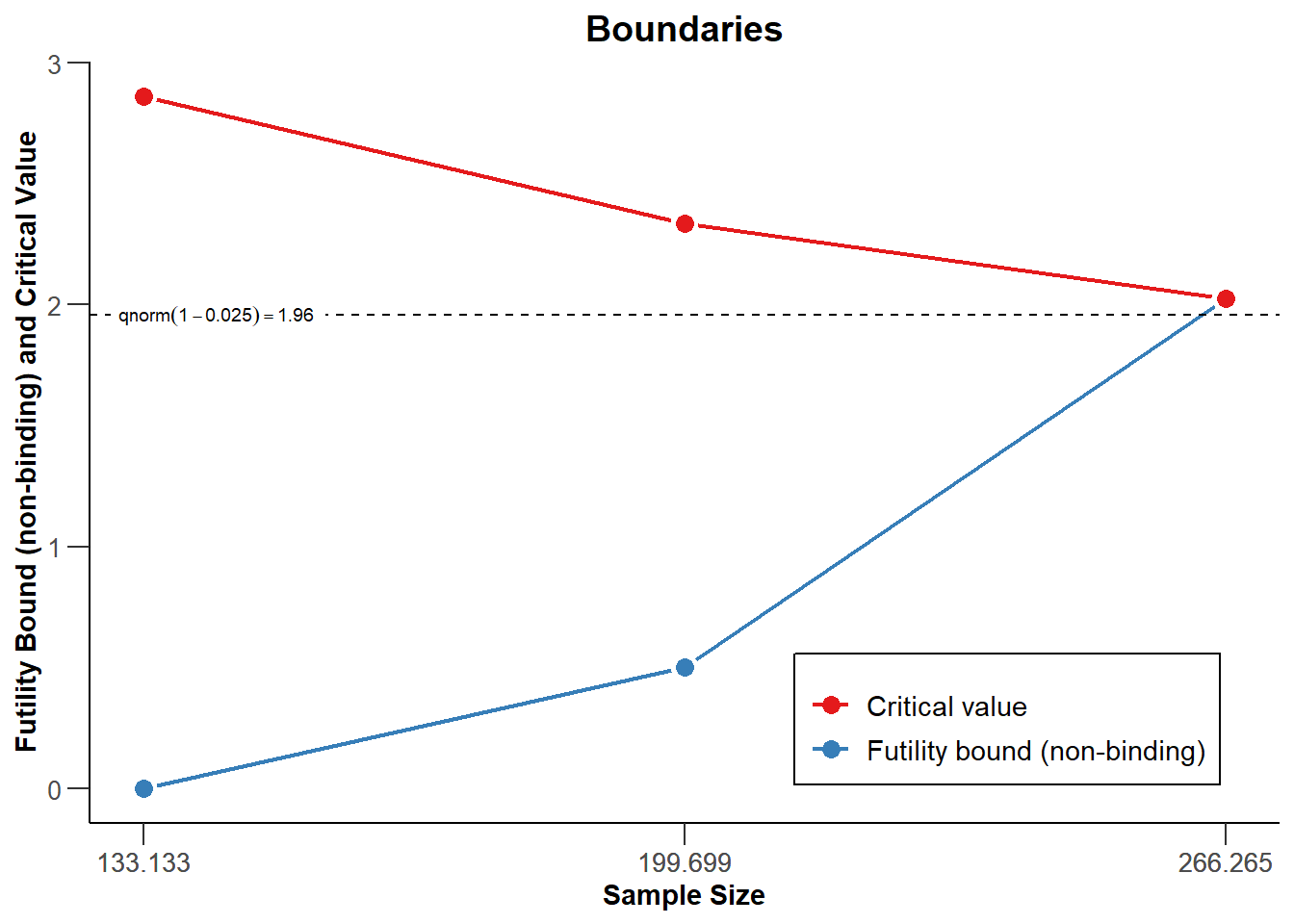On the effect size scale:

plot(r, type = 2)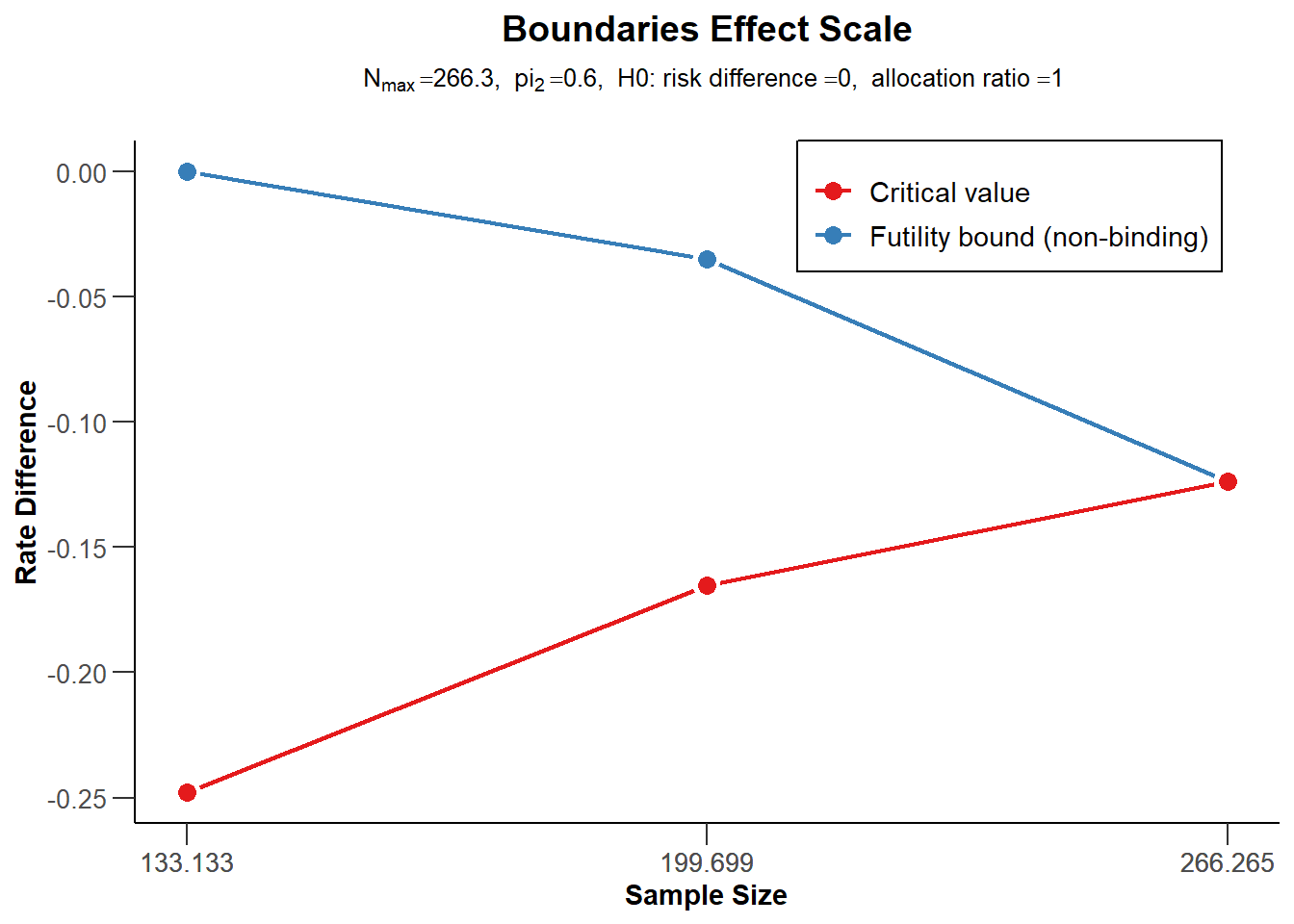On the $$p$$-value scale:

plot(r, type = 3)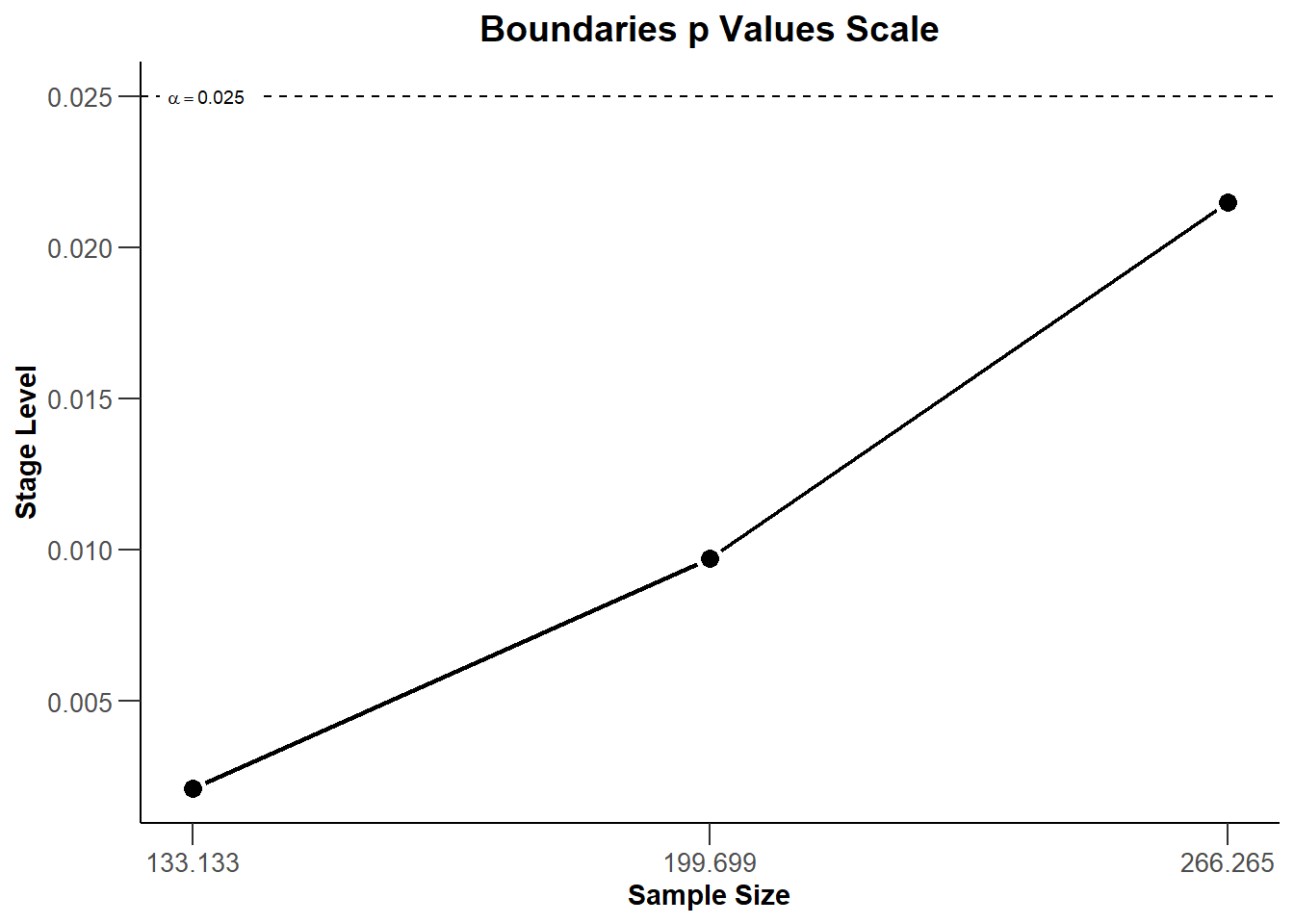## 1.4 Power assessment

Suppose that $$N = 280$$ subjects were planned for the study. The power if the failure rate in the active treatment group is $$\pi_1 = 0.40$$ or $$\pi_1 = 0.50$$ can be achieved as follows:

power <- getPowerRates(dGS, maxNumberOfSubjects = 280, pi1 = c(0.4, 0.5), pi2 = 0.6,
directionUpper = FALSE)

power$overallReject ##  0.914045 0.377853 Note that directionUpper = FALSE is used because the study is powered for alternatives $$\pi_1 - \pi_2$$ being smaller than 0. The power for $$\pi_1 = 0.50$$ (37.8%) is much reduced as compared to the case $$\pi_1 = 0.40$$ (where it exceeds 90%): ## 1.5 Graphical illustration We also can graphically illustrate the power, the expected sample size, and the early stopping and futility stopping probabilities for a range of alternative values. This can be done by specifying the lower and the upper bound for $$\pi_1$$ in getPowerRates() and use the generic plot() command with type = 6: power <- getPowerRates(dGS, maxNumberOfSubjects = 280, pi1 = c(0.3,0.6), pi2 = 0.6, directionUpper = FALSE) plot(power, type = 6)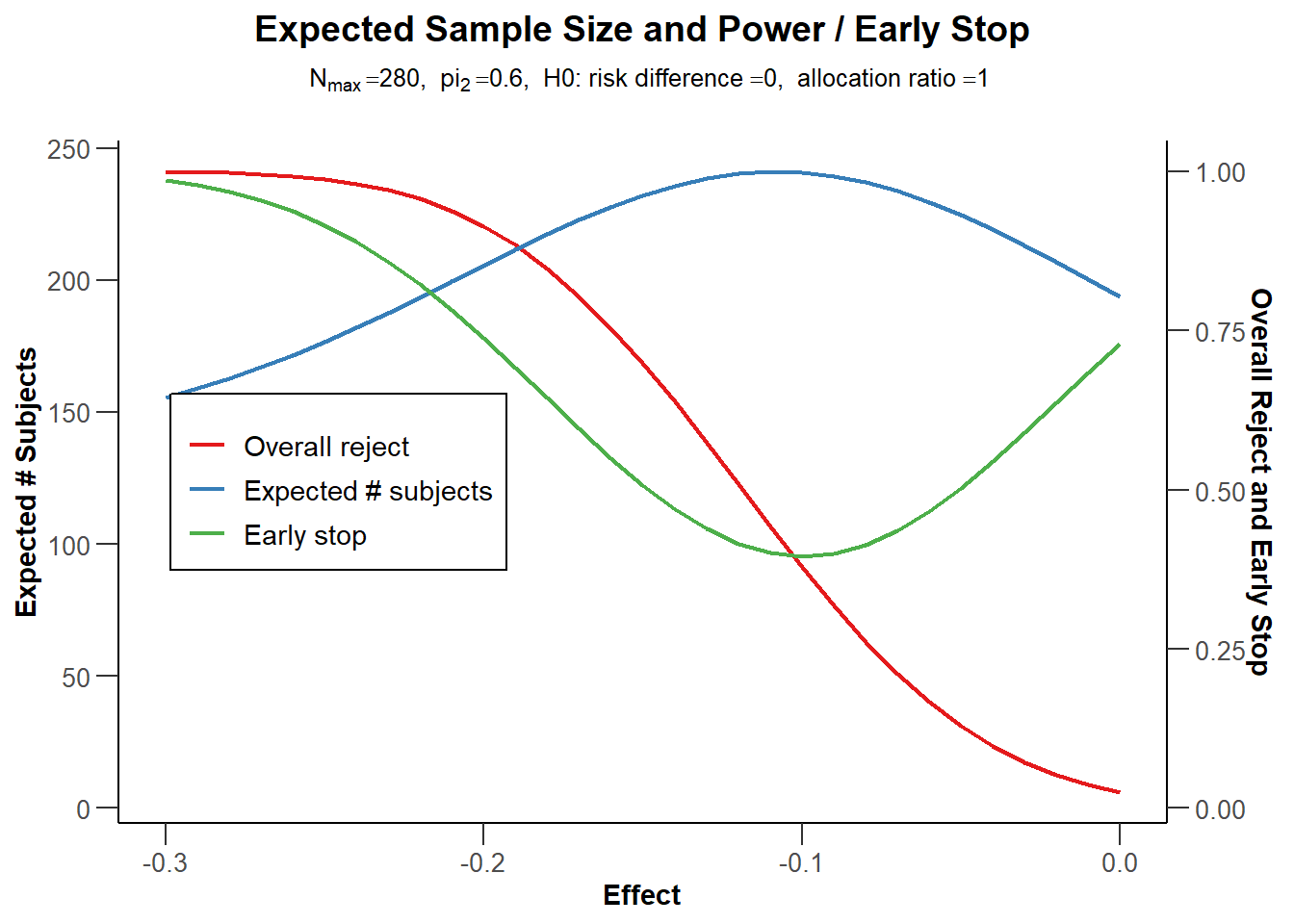# 2 Sample size reassessment for testing rates Suppose that, using an adaptive design, the sample size from the above example can be increased in the last interim up to a 4-fold of the originally planned sample size for the last stage. Conditional power 90% based on the observed effect sizes (failure rates) should be used to increase the sample size. We want to use the inverse normal method to allow for the sample size increase and compare the test characteristics with the group sequential design from the above example. ## 2.1 Assess power To assess the test characteristics of this adaptive design we first define the inverse normal design and then perform two simulations, one without and one with SSR: dIN <- getDesignInverseNormal(informationRates = c(0.5,0.75,1), alpha = 0.025, beta = 0.1, futilityBounds = c(0,0.5)) maxiter <- 1000 seed <- 12345 sim1 <- getSimulationRates(dIN, plannedSubjects = c(140,210,280), pi1 = seq(0.4,0.5,0.01), pi2 = 0.6, directionUpper = FALSE, maxNumberOfIterations = maxiter, conditionalPower = 0.9, minNumberOfSubjectsPerStage = c(140,70,70), maxNumberOfSubjectsPerStage = c(140,70,70), seed = seed) sim2 <- getSimulationRates(dIN, plannedSubjects = c(140,210,280), pi1 = seq(0.4,0.5,0.01), pi2 = 0.6, directionUpper = FALSE, maxNumberOfIterations = maxiter, conditionalPower = 0.9, minNumberOfSubjectsPerStage = c(NA,70,70), maxNumberOfSubjectsPerStage = c(NA,70,4*70), seed = seed) Note that the sample sizes will be calculated under the assumption that the conditional power for the subsequent stage is 90%. If the resulting sample size is larger, the upper bound (4*70 = 280) is used. Note also that sim1 can also be calculated using getPowerRates() or can also easier be simulated without specifying conditionalPower, minNumberOfSubjectsPerStage, and maxNumberOfSubjectsPerStage (which obviously is redundant for sim1) but this way ensures that the calculated objects sim1 and sim2 contain exactly the same parameters and therefore can easier be combined (see below). We can look at the power and the expected sample size of the two procedures and assess the power gain of using the adaptive design which comes along with an increased expected sample size: sim1$pi1
##   0.40 0.41 0.42 0.43 0.44 0.45 0.46 0.47 0.48 0.49 0.50
round(sim1$overallReject,3) ##  0.918 0.897 0.855 0.814 0.761 0.729 0.664 0.578 0.510 0.451 0.390 round(sim2$overallReject,3)
##   0.973 0.965 0.944 0.939 0.881 0.859 0.793 0.739 0.677 0.589 0.514
round(sim1$expectedNumberOfSubjects,1) ##  204.3 207.3 214.3 218.1 225.6 230.9 232.1 235.3 239.1 237.2 237.4 round(sim2$expectedNumberOfSubjects,1)
##   237.8 250.3 263.4 277.4 287.7 307.4 318.2 331.6 337.2 336.8 351.8

## 2.2 Illustrate power difference

We now want to graphically illustrate the gain in power when using the adaptive sample size recalculation. We use ggplot2 (see ggplot2.tidyverse.org and cran.r-project.org/package=ggplot2) for doing this. First, a data set df combining sim1and sim2 is defined with the additional variable SSR. Defining mytheme and using the following ggplot2 commands, the difference in power and ASN of the two strategies is illustrated. It shows that at least for (absolute) effect difference > 0.15 an overall power of more than around 85% can be achieved with the proposed sample size recalculation strategy.

library(ggplot2)

dataSim1 <- as.data.frame(sim1, niceColumnNamesEnabled = FALSE)
dataSim2 <- as.data.frame(sim2, niceColumnNamesEnabled = FALSE)

dataSim1$SSR <- rep("no SSR", nrow(dataSim1)) dataSim2$SSR <- rep("SSR", nrow(dataSim2))
df <- rbind(dataSim1, dataSim2)

myTheme = theme(
axis.title.x = element_text(size = 12), axis.text.x = element_text(size = 12),
axis.title.y = element_text(size = 12), axis.text.y = element_text(size = 12),
plot.title = element_text(size = 14, hjust = 0.5),
plot.subtitle = element_text(size = 12, hjust = 0.5))

p <- ggplot(data = df,
aes(x = effect, y = overallReject, group = SSR, color = SSR)) +
geom_line(size = 1.1) +
geom_line(aes(x = effect, y = expectedNumberOfSubjects / 400,
group = SSR, color = SSR), size = 1.1, linetype = "dashed") +
scale_y_continuous("Power",  sec.axis = sec_axis(~ . * 400, name = "ASN"),
limits = c(0.2, 1)) + xlab("effect") +
ggtitle("Power and ASN", "Power solid, ASN dashed") +
geom_hline(size = 0.5, yintercept = 0.8, linetype = "dotted") +
geom_hline(size = 0.5, yintercept = 0.9, linetype = "dotted") +
geom_vline(size = 0.5, xintercept = c(-0.2, -0.15), linetype = "dashed") +
theme_classic() + myTheme

plot(p)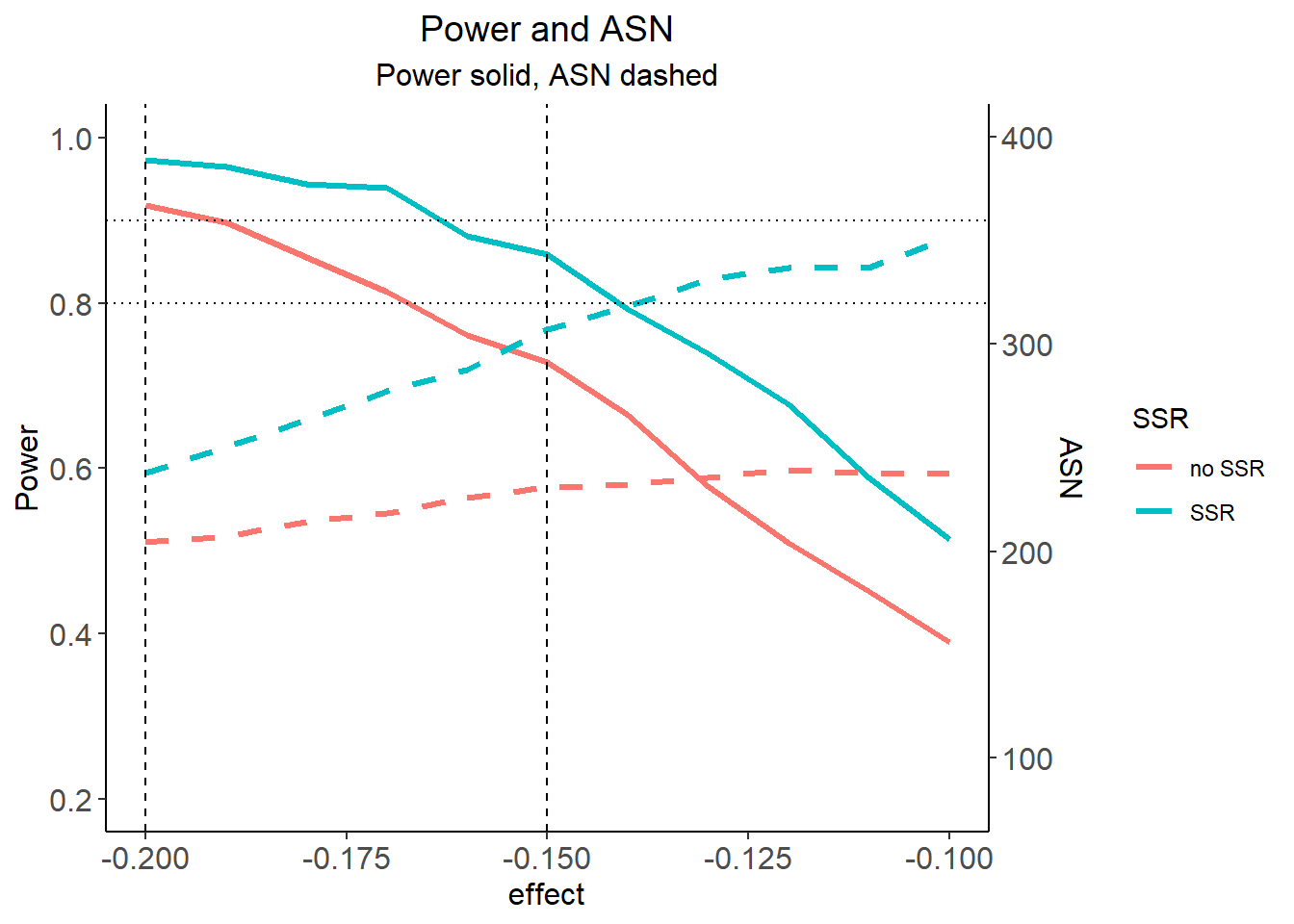For saving the graph, use

ggplot2::ggsave(filename = "c:/yourdirectory/comparison.png", plot = ggplot2::last_plot(), device = NULL, path = NULL, scale = 1.2, width = 20, height = 12, units = "cm", dpi = 600, limitsize = TRUE)

For another example of using ggplot2 in rpact see also this vignette: vignettes.rpact.org/html/rpact_ggplot_examples.html.

## 2.3 Histogram of sample sizes

Finally, we create a histogram for the attained sample size of the study when using the adaptive sample size recalculation.

With the getData() command the simulation results are obtained and str(simdata) provides information of the struture of this data:

simData <- getData(sim2)
str(simData)
## 'data.frame':    24391 obs. of  17 variables:
##  $iterationNumber : num 1 1 1 2 2 3 4 4 5 5 ... ##$ stageNumber             : num  1 2 3 1 2 1 1 2 1 2 ...
##  $pi1 : num 0.4 0.4 0.4 0.4 0.4 0.4 0.4 0.4 0.4 0.4 ... ##$ pi2                     : num  0.6 0.6 0.6 0.6 0.6 0.6 0.6 0.6 0.6 0.6 ...
##  $numberOfSubjects : num 140 70 280 140 70 140 140 70 140 70 ... ##$ rejectPerStage          : num  0 0 1 0 1 1 0 1 0 1 ...
##  $futilityPerStage : num 0 0 0 0 0 0 0 0 0 0 ... ##$ testStatistic           : num  1.18 1.11 2.03 0.34 2.35 ...
##  $testStatisticsPerStage : num 1.184 0.239 2.153 0.34 3.592 ... ##$ overallRates1           : num  0.429 0.438 0.449 0.429 0.371 ...
##  $overallRates2 : num 0.529 0.514 0.555 0.457 0.533 ... ##$ stagewiseRates1         : num  0.429 0.457 0.457 0.429 0.257 ...
##  $stagewiseRates2 : num 0.529 0.486 0.586 0.457 0.686 ... ##$ sampleSizesPerStage1    : num  70 70 70 70 70 70 70 70 70 70 ...
##  $sampleSizesPerStage2 : num 70 70 70 70 70 70 70 70 70 70 ... ##$ trialStop               : logi  FALSE FALSE TRUE FALSE TRUE TRUE ...
##  $conditionalPowerAchieved: num NA 0.061313 0.19483 NA 0.000438 ... Depending on $$\pi_1$$ (in this example, for $$\pi_1 = 0.5$$), you can create the histogram of the simulated total sample size as follows: simDataPart <- simData[simData$pi1 == 0.5,]

overallSampleSizes <-
sapply(1:maxiter, function(i) {
sum(simDataPart[simDataPart$iterationNumber == i,]$numberOfSubjects)
})

hist(overallSampleSizes, main = "Histogram", xlab = "Achieved sample size")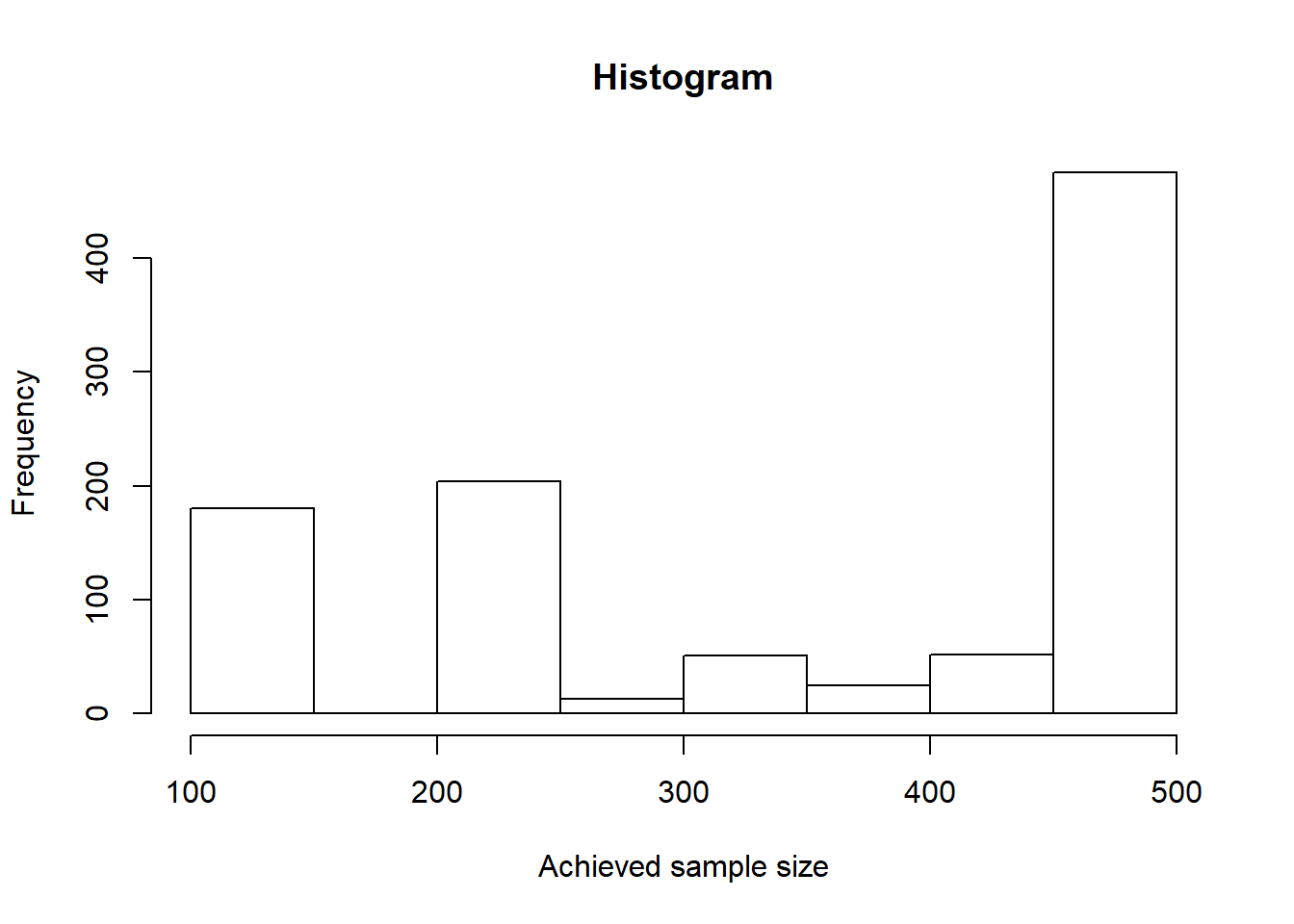How often the maximum and other sample sizes are reached over the stages can be obtained as follows:

subjectsRange <- cut(simDataPart$numberOfSubjects, c(69, 70, 139, 140, 210, 279, 280)) round(prop.table(table(simDataPart$stageNumber,subjectsRange), margin = 1)*100,1)
##    subjectsRange
##     (69,70] (70,139] (139,140] (140,210] (210,279] (279,280]
##   1     0.0      0.0     100.0       0.0       0.0       0.0
##   2   100.0      0.0       0.0       0.0       0.0       0.0
##   3     0.0     10.1       0.3       7.6       5.5      76.5